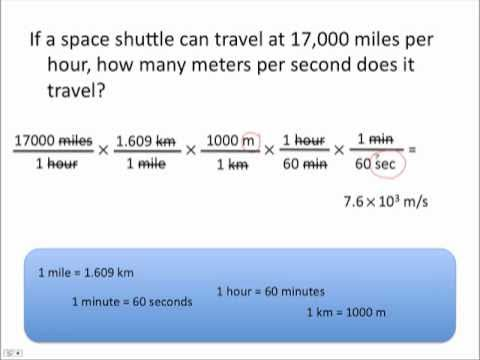# What is an example of a units of measurement practice problem?

Here is an example: A Canadian vacationing in the United States pays $US 3.53/gal for gasoline. What is the equivalent price in Canadian dollars per litre? (1 gal = 3.785 L, and$1 CDN = $0.913 US.) Answer You must convert US dollars → CDN dollars and gallons → litres. $\left(\text{$US "3.53)/(1" gal") × ("$CDN "1)/("$US "0.913) × (1" gal")/(3.785" L}\right)$ = $CDN 1.02/L Notice how the conversion factors are set up to make the units "gal" and "$US" cancel.